String to Number Conversion in Julia

• Last Updated : 25 Aug, 2020

Julia is a flexible, dynamic, and high-level programming language that can be used to write any application. Also, many of its features can be used for numerical analysis and computational science. Julia is being widely used in machine learning, visualization, and data science. Julia allows type conversion for compatible datatypes. Julia has an inbuilt parse method that allows conversion of string to numeric datatype.

A string can be converted to the desired numeric datatype until it’s an invalid string. We can also specify the base for conversion like decimal, binary, octal or hexadecimal.

Syntax: parse(T::Type, str, base=Int)

Parameters:
Type: Specifies the datatype to which the String is to be converted.
str: It is the String that is to be converted to the specified datatype.
base: This is optional. Required only if the String must be converted to a Number of specific bases.

Following are the ways for conversion from String to Number in Julia:

Converting String Input to Number

The string input is taken from the user using the readline() method of Julia. Next, the parse() method is used to convert the String into Integer datatype. The typeof() method outputs the datatype of the resulting integer.

Julia

 # reads the string a = readline()   # prints the string println(a)   # parsing the string to integer print(typeof(parse(Int64, a)))

Output :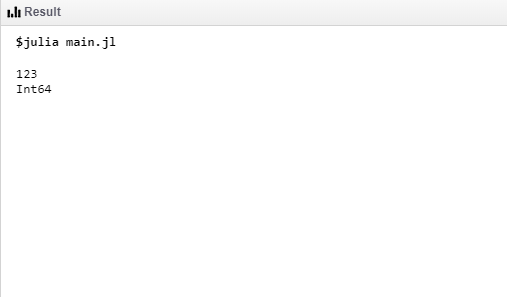Converting String to a Number with some base

The string input is taken from the user using the readline() method of Julia. Next, the parse() method is used to convert the String into Integer datatype with the given base(here octal). The typeof() method outputs the datatype of the resulting integer.

Julia

 # Taking User Inputprintln("Enter the number") a = readline() println("Entered the number: "a)   # 8 is the octal base println(parse(Int64, a, 8))   # Getting Type of dataprint(typeof(parse(Int64, a, 8)))

Output :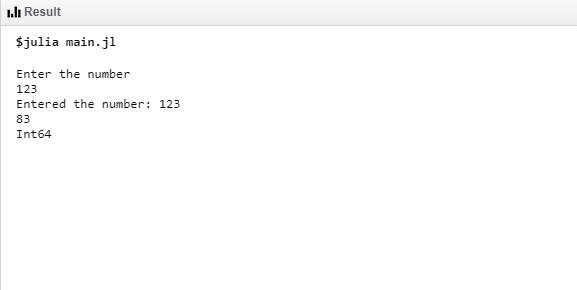Taking String Input and base from the user and converting it into Number

The string input and required base are taken from the user using the readline() method of Julia. The parse() method converts the base into Integer(Int64). Next, the parse() method is used to convert the String into Integer datatype with the given base(here hexadecimal). The typeof() method outputs the datatype of the resulting integer.

Julia

 # Taking String from userprintln("Enter the number") a = readline() println("Entered the number: "a)   # Taking base from userprintln("Enter the base(10,8,16,2)") base = readline() println("Entered base: "base)   # Converting to Numberprintln(parse(Int64, a, parse(Int64,base))) print(typeof(parse(Int64, a, parse(Int64,base))))

Output :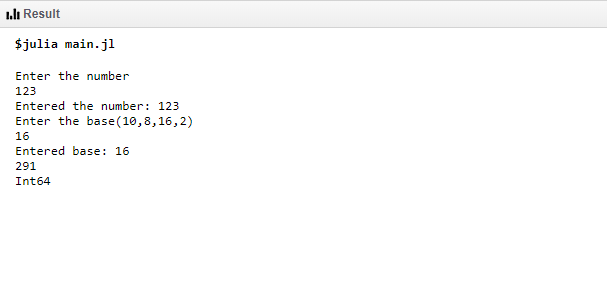Convert String to Float64

The string input is taken from the user using the readline() method of Julia. Next, the parse() method is used to convert the String into Float datatype. The typeof() method outputs the datatype of the resulting float value.

Julia

 # parsing string into float println(parse(Float64, "123.345")) print(typeof(parse(Float64, "123.345")))

Output :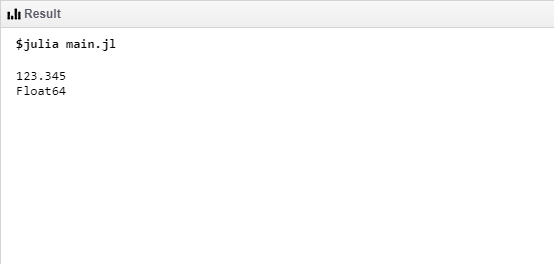Invalid String throws an exception

Since the given string cannot be converted into Float type the parse() method throws an error.

Julia

 # error will be thrown as string# is not a valid float representation print(parse(Float64, "123.345a"))

Output :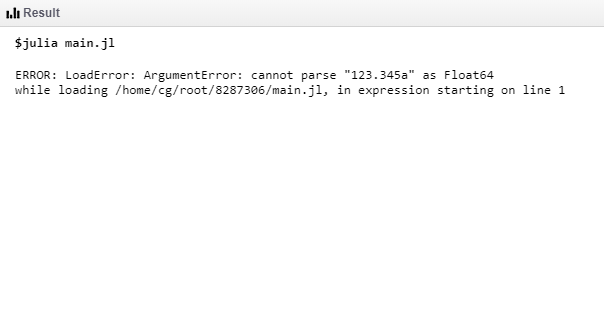My Personal Notes arrow_drop_up